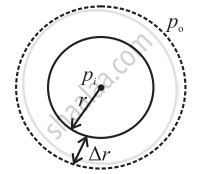# Derive an Expression for Excess Pressure Inside a Drop of Liquid. - Physics

Sum

Derive an expression for excess pressure inside a drop of liquid.

#### SolutionConsider a spherical drop as shown in the figure. Let pi be the pressure inside the drop and p0 be the pressure out side it. As the drop is spherical in shape, the pressure, pi, inside the drop is greater than p0, the pressure outside. Therefore, the excess pressure inside the drop is pi - p0.

Let the radius of the drop increase from r to r + ∆r, where ∆r is very small, so that the pressure inside the drop remains almost constant.

Let the initial surface area of the drop be A1 = 4πr2 , and the final surface area of the drop be A2 = 4π (r+∆r)2.

∴ A2 = 4π(r2 + 2r∆r + ∆r2)

∴ A2 = 4πr2 + 8πr∆r + 4π∆r2 (As ∆r is very small, ∆r2 can be neglected)

∴ A2 = 4πr2 + 8πr∆r

Thus, increase in the surface area of the drop is

dA = A_2 – A_1 = 8πrDeltar

Work done in increasing the surface area by dA is stored as excess surface energy.

therefore dW = TdA= T (8πrDeltar) ... (1)

This work done is also equal to the product of the force F which causes increase in the area of the drop and the displacement ∆r which is the increase in the radius of the bubble.

therefore dW = F∆r ... (2)

The excess force is given by,

(Excess pressure) ×(Surface area)

∴ F = (pi – p0) 4πr2 ... (3)

Equating Eq. (1), and Eq. (2), we get,

T(8πrDeltar) = FDeltar

therefore T(8πrDeltar) = (p_i – p_0) 4πr^2Deltar ... (using Eq. (3))

therefore (p_i – p_0)=(2T)/r

This equation gives the excess pressure inside a drop of liquid.

Concept: Surface Tension
Is there an error in this question or solution?
Chapter 2: Mechanical Properties of Fluids - Exercises [Page 54]

#### APPEARS IN

Balbharati Physics 12th Standard HSC Maharashtra State Board
Chapter 2 Mechanical Properties of Fluids
Exercises | Q 6 | Page 54

Share